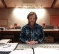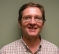## Calculating optimal loop point in audio sampleStarted by 11 months ago10 replieslatest reply 10 months ago181 views

(Disclaimer, I'm self-taught and mostly a beginner when it comes to DSP)

Hi! I've recently been working on a problem to find the optimal loop point in an audio sample, specifically a pipe organ sample. The idea is to find two points in the audio that there will be a seamless jump, completely inaudible.

My current approach is brute-force. I pick an arbitrary loop end point, create a vector with the audio right before and right after the loop, and then calculate the harmonic distortion of that vector. I then find the loop point that causes the smallest amount of distortion.

Unfortunately, it doesn't work, and I'm scratching my head a bit. If you listen to the generated loop, it's quite jarring. I'm trying to identify whether my scoring function is off, or whether I'm going the wrong direction completely.

Here's an excerpt from the code that calculated the score (python):

def calc_harmonics(x):
return abs(rfft(x))
# where to find the loop point in
loop_search_slice = nontremmed[floor(attack_index):floor(release_index)]

# slice_width is how wide of a slice to take from the beginning and end of the loop
# align it to a power of 2 for FFT
slice_width = 2 ** ceil(log2(max((sample_rate / freq), 512)))

# ref_sample is the reference sample (for normalizing test_loop)
ref_sample = loop_search_slice[0:slice_width]
ref_sample_amps = resample_to(calc_harmonics(ref_sample), slice_width + 1)

# this is a curve that biases the lower frequencies, making them more punishing
harmonic_bias = (1 - (np.linspace(0.0, 1.0, slice_width + 1) ** 3)) * 2

lowest_score = math.inf
lowest_index = -1

for i in np.arange(len(loop_search_slice) * 0.6, len(loop_search_slice) - slice_width, 2):
pos = floor(i)

# calculate score based on what provides the least harmonic distortion
potential_end = loop_search_slice[(pos - slice_width):pos]

# loop to test distortion
test_loop = np.concatenate((potential_end, ref_sample))
test_loop_amps = calc_harmonics(test_loop)

# normalize the amplitudes by the reference sample
test_loop_amps_norm = test_loop_amps / ref_sample_amps

# bias the lower frequencies, they are more audible
test_loop_amps_biased = np.maximum(test_loop_amps_norm, ref_sample_amps) * harmonic_bias

# calculate distortion (sqrt isn't necessary)
score = np.sum(np.abs(test_loop_amps_biased)**2)

if score < lowest_score:
lowest_score = score
lowest_index = pos


I have all the code hosted here, the file that does the analysis is envelope.py. Any help would be appreciated!

[ - ]Ideally, the loop length would be an integer number of wave cycles. Allow for some overlap of the start and end, and choose the length such that the overlapping ends have highest possible similarity (i.e. correlation). Then crossfade between end and start, as others have suggested. You might have to equalize volume across the loop, althoung it should not be much of an issue for organ pipes.

[ - ]Hi,

I would suggest you apply slew on the boundary and then simply concatenate. Slew shall be a dissenting ramp and end and  ascending ramp at the beginning.

In most cases,  a slew of 256 samples slew should give inaudible distortion. You can shorten the slew duration if you can afford to use some non-linear slew instead of ramp, like Blackman window portions.

Hope it helps.

Chalil

[ - ]Hi, thank you! I had considered this, but my biggest concern is it would no longer be in phase.

I could be wrong on this, but I think in my case phase it very important. An example of why: in an organ, I have more than one pipe engaged per key. A common combination is called a "celeste." A celeste pipe is tuned slightly sharp relative to the others, so there are slow beats. If I just cross-faded the loop, I expect those beats would be jarred.

[ - ]In audio editing application we make the edit based on the visual waveform (zoom right into the wave sample) at the point where the wave crosses 0 on the vertical axis. Then make sure the next edit wave come to together in similar wave shape - meaning if the wave cycle was going down to 0 so then the edited next section of wave continues on down below 0.

[ - ]This is an interesting approach. In the case of automation, how would I determine which intersection of 0 was the beginning of the wave? Could I check with the dot product?

Previously I tried an approach that used the dot product to determine whether two sections of audio would work to loop, but it never really worked. Granted, I didn't try the approach of starting at 0, I'll have to try it again with that modification.

While I was working on the dot product approach, I started paying attention to how I checked whether a loop was good or not, and I realized I was listening for a click. I thought I'd be able to determine what an audible click was based on harmonic distortion, but that hasn't worked either.

[ - ]This is an interesting problem that is seen by people who operate an audio mixer system. You are trying to match continuity of amplitude and all orders of derivatives of two signals at their boundaries. Tough problem but solved many times in audio systems... you form a fade envelope which gently slides amplitude towards zero as you move from one input to another envelope that gently moves from zero to maximum amplitude and form the sum of the two fade weighted envelopes. It always works... we used to do this in a shaker control system that continuously changed driving signal to obtain a desired response signal spectrum. The boundary conditions of the algorithm persisted in being audible as the control system manipulated the signal in successive blocks. The down fade and up fade envelopes, we called them onions, completely hid the boundary conditions. the shaker being a very fancy loud speaker!

fred h

[ - ]Hi,

Maybe I don't get your goal but I assume it is producing a continuous sound of a sample of organ sound. Why don't you take exactly one period of the fundamental and recirculate it? If you take both upgoing zero crossings or both downgoing zero crossing you'll get a perfect continuous sound.

[ - ]I'm assuming that each of the sounding pipes is independent.  Each of them has a resonance and is walking in phase.  If so I don't see how you can make them continuous in a loop because there is no way to satisfy the multitude of phases.

I was thinking what others have said.  I would try a raised cosine shape for the mixing fall off.  The "trailing" edge is 90 degrees ahead of the "leading" edge.  With a slight shift the sum of the overlapped wave shapes is unity.

[ - ]